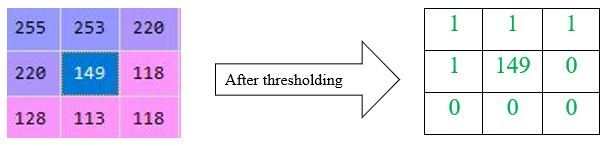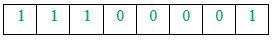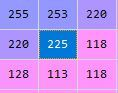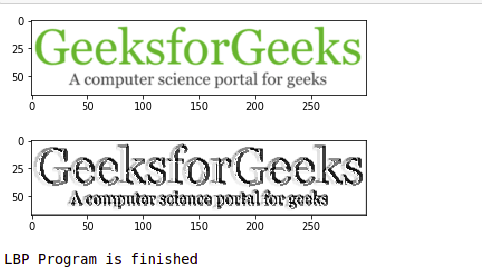# Create Local Binary Pattern of an image using OpenCV-Python

• Difficulty Level : Medium
• Last Updated : 06 Mar, 2020

In this article, we will discuss the image and how to find a binary pattern using the pixel value of the image.

As we all know, image is also known as a set of pixels. When we store an image in computers or digitally, it’s corresponding pixel values are stored. So, when we read an image to a variable using OpenCV in Python, the variable stores the pixel values of the image.

Attention geek! Strengthen your foundations with the Python Programming Foundation Course and learn the basics.

To begin with, your interview preparations Enhance your Data Structures concepts with the Python DS Course. And to begin with your Machine Learning Journey, join the Machine Learning - Basic Level Course

As we can see in following example:

 `import` `cv2``image ``=` `cv2.imread(``"GFG.jpg"``)`` ` `# Now, the variable 'image' stores the pixel values of image``print``(image)`

Examples:

Input :Output :

```[[[255 255 255]
[255 255 255]
[255 255 255]
...
[255 255 255]
[255 255 255]
[255 255 255]]

[[255 255 255]
[255 255 255]
[255 255 255]
...
[255 255 255]
[255 255 255]
[255 255 255]]

[[255 255 255]
[255 255 255]
[255 255 255]
...
[255 255 255]
[255 255 255]
[255 255 255]]

...

[[255 255 255]
[255 255 255]
[255 255 255]
...
[255 255 255]
[255 255 255]
[255 255 255]]

[[255 255 255]
[255 255 255]
[255 255 255]
...
[255 255 255]
[255 255 255]
[255 255 255]]

[[255 255 255]
[255 255 255]
[255 255 255]
...
[255 255 255]
[255 255 255]
[255 255 255]]]```

Pixel values of the image will be stored in the variable and below is a part of the NumPy array which stores the values.

## Local Binary Pattern

There are lots of different types of texture descriptors are used to extract features of an image. Local Binary Pattern, also known as LBP, is a simple and grey-scale invariant texture descriptor measure for classification. In LBP, a binary code is generated at each pixel by thresholding it’s neighbourhood pixels to either 0 or 1 based on the value of the centre pixel.

The rule for finding LBP of an image is as follows:

1. Set a pixel value as center pixel.
2. Collect its neighbourhood pixels (Here I am taking a 3 x 3 matrix so; total number of neighbourhood pixel is 8)
3. Threshold it’s neighbourhood pixel value to 1 if its value is greater than or equal to centre pixel value otherwise threshold it to 0.
4. After thresholding, collect all threshold values from neighbourhood either clockwise or anti-clockwise. The collection will give you an 8-digit binary code. Convert the binary code into decimal.
5. Replace the center pixel value with resulted decimal and do the same process for all pixel values present in image.

Let’s take an example to understand it properly.

Let’s take a pixel value from the above output to find its binary pattern from its local neighbourhood. So, I am taking a value ‘149’ (present at 15th row and 19nd column) and its 8 neighbourhood pixels to form a 3 x 3 matrix.Collect the thresholding values either clockwise or anti-clockwise. Here, I am collecting them clockwise from top-left. So, after collecting, the binary value will be as follows:Then, convert the binary code into decimal and place it at center of matrix.

```1 x 27 + 1 x 26 + 1 x 25 + 0 x 24 + 0 x 23 + 0 x 22 + 0 x 21 +1 x 20
= 128 + 64 + 32 + 0 + 0 + 0 + 0 + 1
= 225```

Now, the resulted matrix will look like,Now, let’s do it using python

 `import` `cv2``import` `numpy as np``from` `matplotlib ``import` `pyplot as plt``  ` `     ` `def` `get_pixel(img, center, x, y):``     ` `    ``new_value ``=` `0``     ` `    ``try``:``        ``# If local neighbourhood pixel ``        ``# value is greater than or equal``        ``# to center pixel values then ``        ``# set it to 1``        ``if` `img[x][y] >``=` `center:``            ``new_value ``=` `1``             ` `    ``except``:``        ``# Exception is required when ``        ``# neighbourhood value of a center``        ``# pixel value is null i.e. values``        ``# present at boundaries.``        ``pass``     ` `    ``return` `new_value``  ` `# Function for calculating LBP``def` `lbp_calculated_pixel(img, x, y):``  ` `    ``center ``=` `img[x][y]``  ` `    ``val_ar ``=` `[]``     ` `    ``# top_left``    ``val_ar.append(get_pixel(img, center, x``-``1``, y``-``1``))``     ` `    ``# top``    ``val_ar.append(get_pixel(img, center, x``-``1``, y))``     ` `    ``# top_right``    ``val_ar.append(get_pixel(img, center, x``-``1``, y ``+` `1``))``     ` `    ``# right``    ``val_ar.append(get_pixel(img, center, x, y ``+` `1``))``     ` `    ``# bottom_right``    ``val_ar.append(get_pixel(img, center, x ``+` `1``, y ``+` `1``))``     ` `    ``# bottom``    ``val_ar.append(get_pixel(img, center, x ``+` `1``, y))``     ` `    ``# bottom_left``    ``val_ar.append(get_pixel(img, center, x ``+` `1``, y``-``1``))``     ` `    ``# left``    ``val_ar.append(get_pixel(img, center, x, y``-``1``))``      ` `    ``# Now, we need to convert binary``    ``# values to decimal``    ``power_val ``=` `[``1``, ``2``, ``4``, ``8``, ``16``, ``32``, ``64``, ``128``]``  ` `    ``val ``=` `0``     ` `    ``for` `i ``in` `range``(``len``(val_ar)):``        ``val ``+``=` `val_ar[i] ``*` `power_val[i]``         ` `    ``return` `val``  ` `path ``=` `'GFG.png'``img_bgr ``=` `cv2.imread(path, ``1``)``  ` `height, width, _ ``=` `img_bgr.shape``  ` `# We need to convert RGB image ``# into gray one because gray ``# image has one channel only.``img_gray ``=` `cv2.cvtColor(img_bgr,``                        ``cv2.COLOR_BGR2GRAY)``  ` `# Create a numpy array as ``# the same height and width ``# of RGB image``img_lbp ``=` `np.zeros((height, width),``                   ``np.uint8)``  ` `for` `i ``in` `range``(``0``, height):``    ``for` `j ``in` `range``(``0``, width):``        ``img_lbp[i, j] ``=` `lbp_calculated_pixel(img_gray, i, j)`` ` `plt.imshow(img_bgr)``plt.show()``  ` `plt.imshow(img_lbp, cmap ``=``"gray"``)``plt.show()``  ` `print``(``"LBP Program is finished"``)`

Output:The output shown in examples contains some values in its X-axis and Y-axis which refers to the width and height of the input image respectively.

My Personal Notes arrow_drop_up